## Annex60.Utilities.Time

Package with models for time

### Information

This package contains models for time.

Extends from Modelica.Icons.Package (Icon for standard packages).

### Package Content

Name Description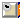CalendarTime Computes the unix time stamp and calendar time from the simulation time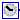ModelTime Model time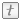Types Package with type definitions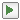Examples Collection of models that illustrate model use and test modelsValidation Collection of models that validate the time models

##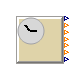Annex60.Utilities.Time.CalendarTime

Computes the unix time stamp and calendar time from the simulation time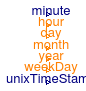### Information

This blocks computes the unix time stamp, date and time and the day of the week based on the Modelica variable `time`.

#### Main equations

First the unix time stamp corresponding to the current time is computed. From this variables the corresponding, year, date and time are computed using functions such as `floor()` and `ceil()`.

#### Assumption and limitations

The implementation only supports date computations from year 2010 up to and including 2020. Daylight saving and time zones are not supported.

#### Typical use and important parameters

The user must define which time and date correspond to `time = 0` using the model parameters `zerTim`, and, if `zerTim==Annex60.Utilities.Time.Types.ZeroTime.Custom`, the parameter `yearRef`. The user can choose from new year, midnight for a number of years: 2010 to 2020 and also 1970. The latter corresponds to a unix stamp of 0. (Note that when choosing the reference time equal to 0 at 1970, the actual simulation time must be within the 2010-2020 range. For instance `startTime = 1262304000` corresponds to the simulation starting on the 1st of January 2010 when setting `zerTim = ZeroTime.UnixTimeStamp`. This is within the 2010-2020 range and is therefore allowed.)

#### Implementation

The model was implemented such that no events are being generated for computing the minute of the day. The model also contains an implementation for setting `time=0` for any day and month other than January first. This is however not activated in the current model since these options may wrongly give the impression that it changes the time based on which the solar position is computed and TMY3 data are read.

Extends from Modelica.Blocks.Icons.DiscreteBlock (Graphical layout of discrete block component icon).

### Parameters

TypeNameDefaultDescription
ZeroTimezerTim Enumeration for choosing how reference time (time = 0) should be defined
IntegeryearRef2016Year when time = 0, used if zerTim=Custom
Timeoffset0Offset that is added to 'time', may be used for computing time in different time zone [s]

### Connectors

TypeNameDescription
output RealOutputunixTimeStampUnix time stamp at local time [s]
output IntegerOutputyearYear
output IntegerOutputmonthMonth of the year
output IntegerOutputdayDay of the month
output IntegerOutputhourHour of the day
output RealOutputminuteMinute of the hour
output IntegerOutputweekDayInteger output representing week day (monday = 1, sunday = 7)

### Modelica definition

model CalendarTime "Computes the unix time stamp and calendar time from the simulation time" extends Modelica.Blocks.Icons.DiscreteBlock; parameter Annex60.Utilities.Time.Types.ZeroTime zerTim "Enumeration for choosing how reference time (time = 0) should be defined"; parameter Integer yearRef(min=firstYear, max=lastYear) = 2016 "Year when time = 0, used if zerTim=Custom"; parameter Modelica.SIunits.Time offset = 0 "Offset that is added to 'time', may be used for computing time in different time zone"; Modelica.Blocks.Interfaces.RealOutput unixTimeStamp(final unit="s") "Unix time stamp at local time"; discrete Modelica.Blocks.Interfaces.IntegerOutput year "Year"; discrete Modelica.Blocks.Interfaces.IntegerOutput month "Month of the year"; Modelica.Blocks.Interfaces.IntegerOutput day(fixed=false) "Day of the month"; Modelica.Blocks.Interfaces.IntegerOutput hour(fixed=false) "Hour of the day"; Modelica.Blocks.Interfaces.RealOutput minute "Minute of the hour"; Modelica.Blocks.Interfaces.IntegerOutput weekDay(fixed=false) "Integer output representing week day (monday = 1, sunday = 7)"; protected final constant Integer firstYear = 2010 "First year that is supported, i.e. the first year in timeStampsNewYear[:]"; final constant Integer lastYear = firstYear + size(timeStampsNewYear,1) - 1; constant Real timeStampsNewYear = { 1262304000, 1293840000, 1325376000, 1356998400, 1388534400, 1420070400, 1451606400, 1483228800, 1514764800, 1546300800, 1577836800, 1609459200} "Epoch time stamps for new years day 2010 to 2021"; constant Boolean isLeapYear = { false, false, true, false, false, false, true, false, false, false, true} "List of leap years starting from firstYear (2010), up to and including 2020"; final constant Integer dayInMonth = {31, 28, 31, 30, 31, 30, 31, 31, 30, 31, 30, 31} "Number of days in each month"; parameter Modelica.SIunits.Time timOff(fixed=false) "Time offset"; // final parameters since the user may wrongly assume that this model shifts the // actual time of the simulation final constant Integer monthRef(min=1, max=12) = 1 "Month when time = 0"; final constant Integer dayRef(min=1, max=31) = 1 "Day when time = 0"; Integer daysSinceEpoch(fixed=false) "Number of days that passed since 1st of January 1970"; discrete Integer yearIndex "Index of the current year in timeStampsNewYear"; discrete Real epochLastMonth "Unix time stamp of the beginning of the current month"; final parameter Modelica.SIunits.Time hourSampleStart(fixed=false) "Time when the sampling every hour starts"; final parameter Modelica.SIunits.Time daySampleStart(fixed=false) "Time when the sampling every day starts"; Boolean hourSampleTrigger "True, if hourly sample time instant"; Boolean daySampleTrigger "True, if daily sample time instant"; Boolean firstHourSampling(fixed=true, start=true) "=true if the hour is sampled the first time"; Boolean firstDaySampling(fixed=true, start=true) "=true if the day is sampled the first time"; initial equation hourSampleStart = integer(time/3600)*3600; daySampleStart = integer(time/(3600*24))*3600*24; hour = integer(floor(rem(unixTimeStamp,3600*24)/3600)); daysSinceEpoch = integer(floor(unixTimeStamp/3600/24)); day = integer(1+floor((unixTimeStamp-epochLastMonth)/3600/24)); weekDay = integer(rem(4+daysSinceEpoch-1,7)+1); initial algorithm // check if yearRef is in the valid range assert(not zerTim == Annex60.Utilities.Time.Types.ZeroTime.Custom or yearRef>=firstYear and yearRef<=lastYear, "The value you chose for yearRef (=" + String(yearRef) + ") is outside of the validity range of " + String(firstYear) + " to " + String(lastYear) + "."); // check if the day number exists for the chosen month and year assert(not zerTim == Annex60.Utilities.Time.Types.ZeroTime.Custom or dayInMonth[monthRef] + (if monthRef==2 and isLeapYear[yearRef-firstYear + 1] then 1 else 0) >=dayRef, "The day number you chose is larger than the number of days contained by the month you chose."); // compute the offset to be added to time based on the parameters specified by the user if zerTim == Annex60.Utilities.Time.Types.ZeroTime.UnixTimeStamp then timOff :=0; elseif zerTim == Annex60.Utilities.Time.Types.ZeroTime.NY2010 or zerTim == Annex60.Utilities.Time.Types.ZeroTime.Custom and yearRef == 2010 then timOff :=timeStampsNewYear; elseif zerTim == Annex60.Utilities.Time.Types.ZeroTime.NY2011 or zerTim == Annex60.Utilities.Time.Types.ZeroTime.Custom and yearRef == 2011 then timOff :=timeStampsNewYear; elseif zerTim == Annex60.Utilities.Time.Types.ZeroTime.NY2012 or zerTim == Annex60.Utilities.Time.Types.ZeroTime.Custom and yearRef == 2012 then timOff :=timeStampsNewYear; elseif zerTim == Annex60.Utilities.Time.Types.ZeroTime.NY2013 or zerTim == Annex60.Utilities.Time.Types.ZeroTime.Custom and yearRef == 2013 then timOff :=timeStampsNewYear; elseif zerTim == Annex60.Utilities.Time.Types.ZeroTime.NY2014 or zerTim == Annex60.Utilities.Time.Types.ZeroTime.Custom and yearRef == 2014 then timOff :=timeStampsNewYear; elseif zerTim == Annex60.Utilities.Time.Types.ZeroTime.NY2015 or zerTim == Annex60.Utilities.Time.Types.ZeroTime.Custom and yearRef == 2015 then timOff :=timeStampsNewYear; elseif zerTim == Annex60.Utilities.Time.Types.ZeroTime.NY2016 or zerTim == Annex60.Utilities.Time.Types.ZeroTime.Custom and yearRef == 2016 then timOff :=timeStampsNewYear; elseif zerTim == Annex60.Utilities.Time.Types.ZeroTime.NY2017 or zerTim == Annex60.Utilities.Time.Types.ZeroTime.Custom and yearRef == 2017 then timOff :=timeStampsNewYear; elseif zerTim == Annex60.Utilities.Time.Types.ZeroTime.NY2018 or zerTim == Annex60.Utilities.Time.Types.ZeroTime.Custom and yearRef == 2018 then timOff :=timeStampsNewYear; elseif zerTim == Annex60.Utilities.Time.Types.ZeroTime.NY2018 or zerTim == Annex60.Utilities.Time.Types.ZeroTime.Custom and yearRef == 2019 then timOff :=timeStampsNewYear; elseif zerTim == Annex60.Utilities.Time.Types.ZeroTime.NY2018 or zerTim == Annex60.Utilities.Time.Types.ZeroTime.Custom and yearRef == 2020 then timOff :=timeStampsNewYear; else timOff :=0; // this code should not be reachable assert(false, "No valid ZeroTime could be identified. This is a bug, please submit a bug report."); end if; // add additional offset when using a custom date and time if zerTim == Annex60.Utilities.Time.Types.ZeroTime.Custom then timOff :=timOff + ((dayRef - 1) + sum({dayInMonth[i] for i in 1:(monthRef - 1)}) + (if monthRef > 2 and isLeapYear[yearRef - firstYear + 1] then 1 else 0))*3600*24; end if; // input data range checks at initial time assert(time + offset + timOff >= timeStampsNewYear, if zerTim == Annex60.Utilities.Time.Types.ZeroTime.UnixTimeStamp then "Could initialize date in the CalendarTime block. You selected 1970 as the time=0 reference. Therefore the simulation startTime must be at least " + String(timeStampsNewYear) + "." elseif zerTim == Annex60.Utilities.Time.Types.ZeroTime.Custom then if yearRef <firstYear then "Could not initialize date in the CalendarTime block. You selected a custom time=0 reference. The minimum value for yearRef is then " + String(firstYear) + " but your value is " + String(yearRef) + "." else "Could not initialize date in the CalendarTime block. You selected a custom time=0 reference. Possibly your startTime is too small." else "Could not initialize date in the CalendarTime block. Possibly your startTime is negative?"); assert(time + offset + timOff < timeStampsNewYear[size(timeStampsNewYear,1)], if zerTim == Annex60.Utilities.Time.Types.ZeroTime.Custom and yearRef >= lastYear then "Could not initialize date in the CalendarTime block. You selected a custom time=0 reference. The maximum value for yearRef is then " + String(lastYear) + " but your value is " + String(yearRef) + "." else "Could not initialize date in the CalendarTime block. Possibly your startTime is too large."); // iterate to find the year at initialization initial algorithm year :=0; for i in 1:size(timeStampsNewYear,1) loop // may be reformulated using break if JModelica fixes bug if unixTimeStamp < timeStampsNewYear[i] and (if i == 1 then true else unixTimeStamp >= timeStampsNewYear[i-1]) then yearIndex :=i - 1; year :=firstYear + i - 2; end if; end for; // iterate to find the month at initialization epochLastMonth := timeStampsNewYear[yearIndex]; month:=13; for i in 1:12 loop if (unixTimeStamp-epochLastMonth)/3600/24 < (if i==2 and isLeapYear[yearIndex] then 1 + dayInMonth[i] else dayInMonth[i]) then // construction below avoids the need of a break, which bugs out JModelica month :=min(i,month); else epochLastMonth :=epochLastMonth + (if i == 2 and isLeapYear[yearIndex] then 1 + dayInMonth[i] else dayInMonth[i])*3600*24; end if; end for; equation // compute unix time step based on found offset unixTimeStamp = time + offset + timOff; // update the year when passing the epoch time stamp of the next year when unixTimeStamp >= timeStampsNewYear[pre(yearIndex)+1] then yearIndex=pre(yearIndex)+1; assert(yearIndex<=size(timeStampsNewYear,1), "Index out of range for epoch vector: timeStampsNewYear needs to be extended beyond the year " + String(firstYear+size(timeStampsNewYear,1))); year = pre(year) + 1; end when; // update the month when passing the last day of the current month when unixTimeStamp >= pre(epochLastMonth) + (if pre(month)==2 and isLeapYear[yearIndex] then 1 + dayInMonth[pre(month)] else dayInMonth[pre(month)])*3600*24 then month = if pre(month) == 12 then 1 else pre(month) + 1; epochLastMonth = pre(epochLastMonth) + (if pre(month)==2 and isLeapYear[yearIndex] then 1 + dayInMonth[pre(month)] else dayInMonth[pre(month)])*3600*24; end when; // compute other variables that can be computed without using when() statements hourSampleTrigger =sample(hourSampleStart, 3600); when hourSampleTrigger then if pre(firstHourSampling) then hour = integer(floor(rem(unixTimeStamp,3600*24)/3600)); else hour = if (pre(hour) == 23) then 0 else (pre(hour) + 1); end if; firstHourSampling = false; end when; daySampleTrigger =sample(daySampleStart, 86400); when daySampleTrigger then if pre(firstDaySampling) then daysSinceEpoch = integer(floor(unixTimeStamp/3600/24)); weekDay=integer(rem(4+daysSinceEpoch-1,7)+1); else daysSinceEpoch = pre(daysSinceEpoch) + 1; weekDay = if (pre(weekDay) == 7) then 1 else (pre(weekDay) + 1); end if; day = integer(1+floor((unixTimeStamp-epochLastMonth)/3600/24)); firstDaySampling = false; end when; // using Real variables and operations for minutes since otherwise too many events are generated minute = (unixTimeStamp/60-daysSinceEpoch*60*24-hour*60); end CalendarTime;

##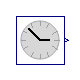Annex60.Utilities.Time.ModelTime

Model time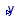### Information

This component outputs the model time, which starts at the value at which the simulation starts. For example, if a simulation starts at t=-1, then this block outputs first t=-1, and its output is advanced at the same rate as the simulation time.

The model is used to allow the simulation to start from any time without having to set the parameters for the clock, as would be necessary for the model Modelica.Blocks.Sources.Clock.

Extends from Modelica.Blocks.Interfaces.SO (Single Output continuous control block).

### Connectors

TypeNameDescription
output RealOutputyConnector of Real output signal

### Modelica definition

block ModelTime "Model time" extends Modelica.Blocks.Interfaces.SO; equation y = time; end ModelTime;

http://iea-annex60.org